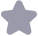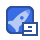### 论文研究-广义离散系统保D-稳定的动态输出反馈.pdf收藏

1854 系统工程理论与实践 第33卷 则系统(3)是正则、因果的,(E,A)cDmn(q,r),且满足‖Gz()<y 证明由(4)的第二个不等式可得(A-gE)P(A-q1)-2E1PE<0,再由EPE≥0,根据引埋2 (E,A)是正则、因果的,且a(E,A)cDnt(q,).利用Sh补引理,由(4)的第二个不等式可得 (A-qE)P(A-QE-rEPE+CC(A-qE)PB+CD <0 BTP(A-qD)+DTC I+DTD+BtPB 令8=e,u∈[0,2丌),对(5)式左乘J B(81E-A1)1r 右乘J,得 (1, 1 )B(sET-A)((A-qETPB+CTD)-22I+DTD+BTPB 0 2,1) -yI+DD+B PB 其中(2,1)可由(6)式的共轭对称性得到,J表示J的共轭转置 1)=(1-q/s-sq)(B"(s-1E-A)-(4PA-EPE)(sE-A)B)+B"PB+BTPA(sE A)-1B+B1(S-1E-A1)-1EPE(sE-A)-1B+B(s-1E1-A1)-1CrC(sE-A)-1B+B E T yTD+ DC(SE-1)B+DTD-22I 注意到,B(s-1E1-A1)-1(APA-EPE)(SE-A)-1B=-BTPB-BTPA(sE-A)-1B T I RT A1)-1ATPB,因此 AT-ETPE(SE-A)B+B(S-E (SE A)B+B(sE-A-CD+DC(sE-A)-B+DD-y2I 再由(6)式得(1,1)<0.由于Dt(y,r)cDnt(0.1),因此q2-r2+1-g/-6>0.这样,由(1,1)<0 得, C-A)CC(SE-A B+B(SE-ACID+DC(sL-AB+DD<2I, 所以,‖G20(z川<7 引理3对于下面矩阵不等式组(7) FXF>O r22ETXE+(A-E+ B2F)X(A-qE+B2F (A-E+B2F)TXB2(A-qE+B2F)XB1(C1+D12FyT ETXE+BXB2 BTXBL ≤0(7) I+BXB 如果存在对称可逆矩阵X满足如下矩阵不等式组: EXE>O 22 Di1D11-B1XB1>0 8 02=FTXE-B2XB2-B2XB1B1 BTX B2>0 ETXE+(A-E)TX(A-gE)+CTC1+05-04c 那么.矩阵F=-03104使得(7)成立.这里“*”代表矩阵元素的对称元素,,是给定的正实数, 03,04,05如(9)所示 03=BXB2+D12D2+月111+(B2XB2+01B1XB2)02(B2XB2+BXB1611) 04=B2X(A-E)+DB2C1+112+(B2XB2+B-1B1XB2)621(BX(A-E)+B2XB10-12) B5=6112+(4-qF)XB2+A61B1XB2)B21(BX(A-qF)+B2XB1B12) (9) 月1=BXB1+5D2D B2=BIX(A-qE)+ 证明由(8)和F--03104,可以得到-2EXE+(A-gE)X(A-qE)+CIC1+05-003104+ (F+634)63(F+0310)≤0.这等价于 r2EXE+(A-qE)X(A-qE)+C1C1+0+p93F+F04+F<0 将(9)代入(10)中,得 r2EXE+(A-gE-B2F)X(A-gE+B2F)+(C1+D12F)(C1+D12F)/2+(4-E+B2F)1XB1 +(C1+D12F)D11/a201[D1(C1+D12 F)/a+BiX(A-qE+ B2F)+[A-qE+B2 F)XB2+ 第7期 贺贺,等:广义离散系统保D-稳定的动态输岀反馈H×控制器设计 1855 ((A-qE+B2F)XB1+(C1+D12F) D11/a2)0, BIXB2](ETXE-BIXB2-B2XB18T BIXB2)- X[B2X(A-qE+B2F)+BXB101(DI(C1+ D12F)a2+BTX(A-qE+B2F))<0 (11) 由(8)、(11)以及Shur补引理可知,矩阵不等式(7)成立,证明完成 矩阵F可以通过求解矩阵不等式组(8)得到 下面,为给出本文的主要结果,我们构造如下辅助广义系统 7Ex(k+1)-(A-g+LC2)()+(B1+LD21j(k) (k) (k) (12) (E XE)/2F 其中L是适当维数矩阵.在已获得矩阵F的前提下,由引理1可知,系统(12)是正则、因果、稳定的,且 G2(2川<6(>0,>0),当且仅当存在如下可逆对称矩阵Y和矩阵L满足 ETYE>O rEYE+(A-qE+LC2)Y(A-qE+ LC2)+FTDTXEF+FTDT D12F/B2 (A-qE+LC2)Y(B1+LD21 B22I+(B1+LD21)Y(B1+LD21 (13) 利用引哩3和(13),我们便可以得到本文的主要结果 定理2对于广义离散系统(1),如果存在可逆对称矩阵X,Y,矩阵F,L以及正实数a,B满足(7),(13) 和α2+β2=1,那么严格真动态输出反馈控制器(2)使得系统(1)是正则、因果的,σ(E,A)<D灬nt(q,r),且 Gz()<,其中控制器(2)的参数为 Ac=A+(B2+LD22F'+ B 证明将控制器(2)代入系统(1),所得闭环系统为: ES(k+1)=Gs()+ Ilw(k 15 x(k)=Js(h)+ Ma(h) 其中, BaCo B E H 0 E Bca Ac+BeDoc BeDel (16) J=[C1,D12C小,M=D11,(k) 根据定理1,闭环系统(15)是正则、因果的,σ(E,D)CDn(q,r),且‖Gzu(z)‖。<,当且仅当存在实 对称可逆矩阵P满足下面矩阵不等式组 FPE>O (G-gETP(G-yE-r2ETPE+J+((G-qETPH +JTM(T MM-HPH)(HP(G-E)+M <0 (17) 1-MM-HPH>O 下面我们证明矩阵P= X+Y -Y 使得(17)成立 容易验证EPE≥0,其中X是矩阵不等式组(8)的解、Y是满足(13)的对称可逆矩阵.将(16)中的 E,G,H,,M,P代入(17)的第二个不等式,由Shu补定理,可得 (1,1)(1,2) 其中,(1)=(4= C B X+Y - Y(A-qE B2C CB T-qET+CTD22BT BC2 Ac-qEtBD22CC n2EPE+cn、 C1D12C|, 1856 系统工程理论与实践 第33卷 (A-qE CoB X+y-Y B AT-qET+CC D 2BT D11 BcD21 )=-2+m1D1+BTD1B X+r -Y B BcD2l X 0 X+y-y 用 替换(18)中的 得到 (A-E)-(A-qE)T+CBT A-gE B2c X 0 其中(1,1)= CrB+At qE+CTDTBT 0 Y-A+qE+B C2 B2Cc + Ac gE+BD22 Cc E E X 0 E 0 D 0E T E p/+ CTDC1CTDD2C」 (A-gE)I T et+caBl q X 0 B D BT+AT-qET+CTDZBT 0 Y-B1+BD T (2,2)=-2I+D1D1+(-B+D21B)Y(-B1+B2D21)+B1XB1 I 0 I00 矩阵(19)左乘010,右乘110,并利用控制器的解析式(14),得 00T 00T (1,1)(1 <0 +CtB2-qE 0 A-qE+B2 B。C 其中(1,1)= CTBT+AT- BcC+Ac- Er+CTDo Br gE+BcD22 C Y0E O C1C1+ Cc D12C1t E10 C1 D12 Cc+C D12D12CC + TC +CTDtD 0 0 Y 0 C1+CDi D12cr CIoD TBT-qET X 0 B 任TDT 2 CTBT+A D B1+BD CD E+CID B TI D1+(BT+D2IBT)Y(B1+BD21)+BTXB 因此只需要证明(20)成立即可由(13)和shur补定理,可得: 0 0 ETYE+(A-gE+LC (A-qE+LC2)+FE XEF (A- qE+LC2)Y(B+LD21) FTDi2 B4yI+(B1+LD21)Y(B1+ LD21) 由(7)和(21),并利用a2+B2=1,有 (21) 0 由上式和Shur补定理得知(20)成立,证明完成 第7期 贺贺,等:广义离散系统保D-稳定的动态输出反馈H∝控制器设计 1857 接下来,我们给出获得矩阵L的方法构造辅助系统(12)的对偶系统 rEx(k +1=((A-qE)+C2I)x(k)+ FTD/6 FT((ETXE)1/2)w(k:) (22) z(k)=(B1+ D2L)c(k 显然满足|G2(2)=‖(G2(2),其中G(x)是系统(12)从u到z的转移函数,G2(2)是系 统(22)从u到z的转移函数 令C-FD卫2/BFT(ETXE)1/2),根据引理1,系统(22是正则因果、稳定的,且|G20(=)‖< 6(3>0.>0),当且仅当存在如下可逆对称矩阵z满足 EZET>O -r2EZE+(A-qE+LC2)Z(A-qE+LC2)+ (B1+LD21)(B1+LD21) (A-qE+LC2)ZC (23) <0 B222+CZC 利用下面的定理叮以获得矩阵L 定理3对于矩阵不等式组(23),如果存在一个对称可逆矩阵Z满足 EZET>O 2222I-CTZC>0 PEZE+(A-qE)Z(A-qE)+BiBT+(A-qE)ZC06 CTZ(A-qE)-0g 9788<0(24) 那么L=-0071使得(23)成立,其中 86=B421-CZC 97=C2ZC+D12D 1+C22C0CTZCT (25) A8=C2Z(A-qET-D12BI+C2ZC06 CTZ(A-qE) 6,Y为给定正数 证明类似于定理1 结论1由定理3,我们不仅得到了满足(23)的矩阵L,而且也获得了设计广义离散系统 2ETc(k+1)=(A-qE)Tc(k)+ FTDT2/B FT((ETXE)1/)w(k:)+C2u(k (26) 2(k)=B1a(k)+D21v(k) 的状态反馈控制器的方法,即控制器u=Lx(k)使系统正则、因果、稳定且‖G2c()‖x<(>0,>0) 结论2定理1,2和3表明当存在可逆对称矩阵Ⅹ,Z满足(⑧)和(24),保证闭环极点位于指定圆域 内的广义离散系统的H控制问题是可解的.而且在这种情况下,严格真动态输出反馈控制器可通过求解两 组矩阵不等式(8)和(24)得到.值得注意的是可解性条件是由系统矩阵表达的,因此设计过程不需要矩阵分 解,可避免由矩阵分解产生的数值问题 结论3对于相对低阶的系统,两组矩阵不等式(⑧)和(24)的求解可以通过数值方法来解决.对于高阶 的系统.求解这些不等式的有效算法仍有待研究 4数值仿真 考虑如下广义离散系统:E 0.50.2 0.150.32 0.20.3 020.1,C 00 0.20.4 0 0.10 B 21 D 0.2 00.5 22 0.1 00.,D1=0,D2=0.010.01 要求H。范数界=1 令α=0.8,=0.6,7=0.8,q=0.1,首先通过解矩阵不等式(8),得 0.4097-0.0278 0.81180.6235 X=1.0e-003 -0.0278-0.4152 所以F=-0314 -0.8118-1.6235 然后通过解矩阵不等式(24).得: Z=1.0e-006× 0.02550.0537 1.2226-12469 所以L=-6 0.05370.0465 1858 系统工程理论与实践 第33卷 因此,最终得到动态输出反馈控制器为: 1.15700.3442 1.22261.2469 Ac=A+(B2+LD22F+LC2= B L=- 0.62470.2494 0 0.5 C= F 0.81180.6235 0.8118-1.6235 而且状态反馈控制器u=L"x(k)使系统(26)正则、因果、稳定且|Gza(z)‖。< 5结束语 本文研究保证广义离散系统D-稳定性的动态输出反馈H。控制器设计问题.给出广义离散系统正贝 因果、D稳定并且满足H。范数约束的充分条件.在此基础上,通过构造辅助广义系统,用两组矩阵不等式 给出系统满足上述条件的动态输岀反馈控制器存在的充分条件,并给出控制器旳解析表达式.同时,也得到 了广义离散系统状态反馈控制器的一种设计方法.由于控制器可解性条件是用系统的系数矩阵表达的,设计 过程并不包含系统矩阵的分解,可避免由矩阵分解带来的数值问题.仿真结果证明了所提出方法的有效性. 参考文献 1 Masubuchi I, Kamit ane Y, Ohara. A, et a.l. Hoo control for descriptor systems: A mat rix inequalities approach[J Automatica.1997.33:669673 2]王岩,张庆灵.不确定广义系统动态输出反馈鲁棒H∞控制器设计[].控制与决策,2002,17(6):948-951 Wang Y, Zhang Q L. Dynamic output fccdback robust Hoo control for uncertain descriptor systomsJ. Control and Decision,2002,17(6):948951 3 Ilaidar A, Boukas E K. Exponential stability of singular systems with multiple time-varying delays[J. Automat ica,2009,45(2):539545. 4 Haidar A, Boukas E K, Xu s, et al. Exponential stability and static output feed back stabilisation of singular time-delay systems with saturating actuators[J. IET Control Theory Applications, 2009, 3(9: 1293-1305 5 Zhang J Xia Y, Boukas E K New approach to Hoo control for Markovian jump singular systems J]. IET Control Theory Applications, 2010. 4(11): 2273-2284 6」王建,霍春宝.不确定广义离散系统的鲁棒H- infinity控制!J.系统工程与电子技术,2005,27(12):2086-2090 Wang J, Huo C B Robust H-infinity control for uncertain singular discrete systems[J. Systems Engineering and Electronics,2005,27(12):20862090 7 Sun X, Zhang Q L, Yang CY, et al. Delay-dependent stability analysis and stabilization of discrete-time singular delay systemsJ. Acta Automatica Sinica, 2010, 36(10): 1477-1483 8 Mei F. Delay-dependent stability analysis for discrete singular systen with tirne-varying delaysJ. Acta Auto matica Sinica,2010,36(5):751-755 9 Sun X, Zhang Q L, Yang CY, et al. Stability analysis and stabilization for discrete-time singular delay systemsJ Journal of Systems Engineering and Electronics, 2011, 223): 482-487 10 Lu R Q, Su H Y: Chu J, et al. A simple approach to robust D-stability analysis for uncertain singular delay systems J. Asian Journal of Control, 2009, 11(4: 411-419 11 Chen S H, Chou J H. Robust D-stability analysis for lincar uncertain discrctc singular systems with state dclayJ Applied mathematics Letters, 2006, 19: 197 205 12 Zhang R, Zhang X, Li F. D-stability and D-stabilization of descriptor discrete time-delay systems with poytopic uncertaintiesCl// Proceeding of 29th Chinese Control Conference, Beijing, China, 2010: 915-920 13 Cui P, Zhang C H, Zhang H S, et al. Indefinite linear quadratic optimal control problem for singular discrete-time system with multiple input delays J. Automatica, 2009, 45(10): 2458-2461 [14 Wu Z G, Park H, Su H Y, et al. Admissibility and dissipativity analysis for discrete-time singular system with mixed time-varying delaysJ. Applied Mathematics and Computation, 2012, 218(13): 7128-7138 15 Mao W J. An LMI approach to D-stability and D-stabilization of linear discrete singular system with state delay J. Applied Mathematics and Computation, 2011, 218(5):1691-17041 16 Xu S, Lam J, Yang C. Robust Hoo control for unccrtain discrete singular systcms with pole placement in a disk[J. Systems &e Control Letters, 2001, 43(2): 85-93 17 Lin J X, Fei S M, Shen J. Admissibility analysis and control synthesis for switched linear singular systemsJ Journal of SysteIns Engineering and ElectroniCs, 2009, 20(5): 1037-1044 18 Lin JX, Fei S M, Shen J Robust stability and stabilization for uncertain discrete-time switched singular systems with time-varying delays. Journal of Systems Engineering and Electronics, 2010, 21(4): 650-657 !李吉祥,武俊峰.不确定离散广义系统的冂稳定鲁棒非脆弱可靠控制门J·中南大学学报:自然科学版,2011,42(6) 1669-1675 LiJ X, Wu J F. D-stable robust non-fragile reliable control for uncertain discrete singular systemsJ. Journal of Central South University: Science and Technology, 2011, 12(6: 1669-1675

...展开详情

抢沙发
一个资源只可评论一次，评论内容不能少于5个字
2019-09-20
weixin_38743506•至尊王者

成功上传501个资源即可获取
关注 私信 TA的资源
上传资源赚积分,得勋章
最新推荐论文研究-广义离散系统保D-稳定的动态输出反馈.pdf 9积分/C币 立即下载
1/7试读已结束，剩余4页未读...

9积分/C币 立即下载 ＞